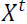1. The minimum length of antenna for efficient transmission of signals of wavelength 𝜆 required is

(a) 𝜆/2
(b) 𝜆/3
(c) 𝜆/4
(d) 𝜆/5

2. In order to describe an algorithm for searching an AND - OR graph, we need to exploit a value called:

(a) Modality
(b) Mobility
(c) Futility
(d) Quality

3. Most commonly used language for Artificial Intelligence programming is:

(a) C
(b) CC++
(c) LISP
(d) PASCAL

4. The process of writing programs that can themselves produce formal descriptions from informal ones is:

(a) Optimization
(b) Feasibility
(c) Coding
(d) Operationalization

5. How well a model trained on the training set predicts the right output for new instances is called:

(a) Specialization
(b) Generalization
(c) Modularization
(d) Optimization

6. In polynomial interpolation, given N points, we find the (N−1)th degree polynomial that is used to predict the output for any X which is outside of the range ofin the training set is called:

(a) Extrapolation
(b) Interpolation
(c) Polynomial evaluation
(d) Friction

7. For which of the following regions, a transistor as a switch will be stable?

(a) Saturation and active
(b) Active and cutoff
(c) Cutoff and saturation
(d) Active and saturation

8. Which of the following is a current controlled device?

(a) JEET
(b) MOSFET
(c) BJT
(d) Zener diode

9. What is the value of an inductance when an inductor filter is connected to a full wave rectifier operating at 60 Hz for providing a dc output voltage with 4 % ripple at 100 Ω load?

(a) 1.5625 𝐻
(b) 2.3525 𝐻
(c) 1.3525 𝐻
(d) 2.5625 𝐻